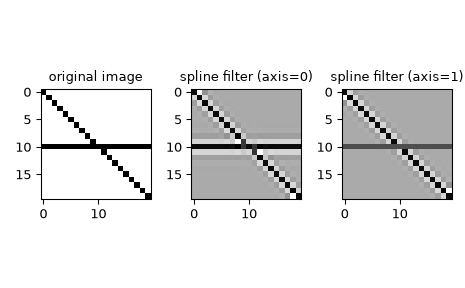# scipy.ndimage.spline_filter1d¶

scipy.ndimage.spline_filter1d(input, order=3, axis=-1, output=<class 'numpy.float64'>, mode='mirror')[source]

Calculate a 1-D spline filter along the given axis.

The lines of the array along the given axis are filtered by a spline filter. The order of the spline must be >= 2 and <= 5.

Parameters
inputarray_like

The input array.

orderint, optional

The order of the spline, default is 3.

axisint, optional

The axis along which the spline filter is applied. Default is the last axis.

outputndarray or dtype, optional

The array in which to place the output, or the dtype of the returned array. Default is numpy.float64.

mode{‘reflect’, ‘constant’, ‘nearest’, ‘mirror’, ‘wrap’}, optional

The mode parameter determines how the input array is extended beyond its boundaries. Default is ‘mirror’. Behavior for each valid value is as follows:

‘reflect’ (d c b a | a b c d | d c b a)

The input is extended by reflecting about the edge of the last pixel.

‘constant’ (k k k k | a b c d | k k k k)

The input is extended by filling all values beyond the edge with the same constant value, defined by the cval parameter.

‘nearest’ (a a a a | a b c d | d d d d)

The input is extended by replicating the last pixel.

‘mirror’ (d c b | a b c d | c b a)

The input is extended by reflecting about the center of the last pixel.

‘wrap’ (a b c d | a b c d | a b c d)

The input is extended by wrapping around to the opposite edge.

Returns
spline_filter1dndarray

The filtered input.

spline_filter

Multidimensional spline filter.

Notes

All functions in ndimage.interpolation do spline interpolation of the input image. If using B-splines of order > 1, the input image values have to be converted to B-spline coefficients first, which is done by applying this 1-D filter sequentially along all axes of the input. All functions that require B-spline coefficients will automatically filter their inputs, a behavior controllable with the prefilter keyword argument. For functions that accept a mode parameter, the result will only be correct if it matches the mode used when filtering.

Examples

We can filter an image using 1-D spline along the given axis:

>>> from scipy.ndimage import spline_filter1d
>>> import matplotlib.pyplot as plt
>>> orig_img = np.eye(20)  # create an image
>>> orig_img[10, :] = 1.0
>>> sp_filter_axis_0 = spline_filter1d(orig_img, axis=0)
>>> sp_filter_axis_1 = spline_filter1d(orig_img, axis=1)
>>> f, ax = plt.subplots(1, 3, sharex=True)
>>> for ind, data in enumerate([[orig_img, "original image"],
...             [sp_filter_axis_0, "spline filter (axis=0)"],
...             [sp_filter_axis_1, "spline filter (axis=1)"]]):
...     ax[ind].imshow(data, cmap='gray_r')
...     ax[ind].set_title(data)
>>> plt.tight_layout()
>>> plt.show()#### Previous topic

scipy.ndimage.spline_filter

#### Next topic

scipy.ndimage.zoom